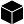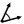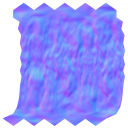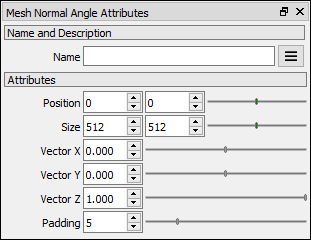# Mesh Normal Angle node

Left Toolbar >Mesh Nodes >* Mesh Normal Angle Node *

The Mesh Normal Angle node renders the angle between the pixel surface normal and a vector defined in the attributes. The Mesh Normal node generates a VALUE image with the angle in radians.

modelsModel

inputimage

outputimage

## Procedure

### To create a Mesh Normal Angle image:

1. Load one of more model in the Scene Window.

2. On the left toolbar choose Mesh Nodes > Mesh Normal Angle.>3. Drag the Mesh Normal Angle icon into the Node Graph.normal

A NORMAL image.

## Outputs

image

A monochromatic image where the white values represent and angle of 0 degrees between the normal and the defined vector, and black is an angle of 180 degrees.

## Attributes PanelPosition Position of the output image.

Size Size of the output image.

Vector X Coordinate x of the vector.

Vector Y Coordinate y of the vector.

Vector Z Coordinate z of the vector.